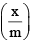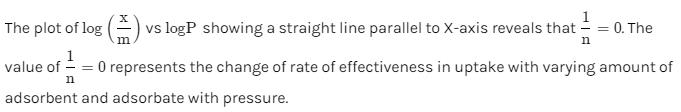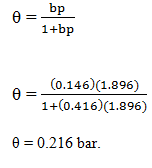Courses

## 12 Questions MCQ Test Physical Chemistry | Test: Adsorption - 1

Description
This mock test of Test: Adsorption - 1 for Chemistry helps you for every Chemistry entrance exam. This contains 12 Multiple Choice Questions for Chemistry Test: Adsorption - 1 (mcq) to study with solutions a complete question bank. The solved questions answers in this Test: Adsorption - 1 quiz give you a good mix of easy questions and tough questions. Chemistry students definitely take this Test: Adsorption - 1 exercise for a better result in the exam. You can find other Test: Adsorption - 1 extra questions, long questions & short questions for Chemistry on EduRev as well by searching above.
QUESTION: 1

### Rate of physisorption increases with:

Solution:

Physisorption is exothermic in nature. Therefore in accordance with Le-Chatliers principle, it decreases with increase in temperature. This means physisorption occurs at a low temperature more readily.

QUESTION: 2

Solution:

Adsorption is accompanied with decrease in entropy and enthalpy of system. The value of  TΔS is negative.

Adsorption decreases randomness and thus, ΔS=−ve and also, TΔS = −ve.

QUESTION: 3

### Which statement is not correct:

Solution:
• Physical adsorption is due to van der Waals forces which are weak forces,
• Physical adsorption decreases at high temperature and low pressure
• Adsorption energy for chemical adsorption is generally more than that for physical adsorption. It is not lesser than that for physical adsorption.
QUESTION: 4

For adsorption of gas on solid surface, the plots of log x/m vs. log P is linear with a slope equal to:

Solution:

For the adsorption of a gas on a solid x/m ​= kp1/n. This equation is known as Freundlich Adsorption Isotherm.

On taking logarithm on both sides, we obtain, logx/m ​= logk+ 1/n​logp
∴ plot of log(x/m) versus log p will be linear with slope = 1/n​ .

QUESTION: 5

Which is correct in case of van der Waals’ adsorption:

Solution:

If the accumulation of gas on the surface of a solid occurs on account of weak van der Waals’ forces, the adsorption is termed as physical adsorption or physisorption.

Hence, Physisorption can also be called as Van der Waals adsorption.

Physical adsorption of a gas by a solid is generally reversible. We can say by Le-Chatelier's principle that more of gas is adsorbed when pressure is increased as the volume of the gas decreases and since the adsorption process is exothermic, the physical adsorption occurs readily at low temperature and decreases with increasing temperature.

So the correct case is when it is Low temperature and high pressure the Physisorption tends to happen.

QUESTION: 6

According to Langmuir adsorption isotherm the amount of gas adsorbed at very high pressure:

Solution:

According to Langmuir Adsorption isotherm, the amount of gas adsorbed at very high pressures reaches a constant limiting value. The amount of gas adsorbed will not increase with the further increase in pressure

QUESTION: 7

Which characteristic is not correct for physical adsorption:

Solution:
• Physical adsorption decreases with an increase in temperature because an increase in temperature leads to an increase in kinetic energy of molecules and hence, their desorption.
• Since physical adsorption is an exothermic process, it occurs more readily at lower temperatures and decreases with an increase in temperature (Le-Chatelier's Principle).
• If the adsorption is a spontaneous phenomenon, it should be exothermic, at least for adsorption from the gaseous phase.
• A molecule in the gas phase will have an entropy higher than in the adsorbed state, then the ΔS should be negative, the only way to have a ΔG negative (spontaneous) is to have a ΔH negative (exothermic process).
• Therefore, the process will be spontaneous (ΔG=−ve) if the temperature is low.
QUESTION: 8

Adsorption of a gas on solid metal surface is spontaneous and exothermic, then:

Solution:
• Adsorption of gas on the solid metal surface is spontaneous and exothermic, then ΔS decreases.
• Since for spontaneous and exothermic process, ΔG = −ve, ΔH = −ve at all temperatures.
• Therefore, from ΔG = ΔH − TΔS,ΔS should be −ve.
• Also, the adsorption of a gas on a solid surface gives a more orderly arrangement.
QUESTION: 9

Surface area available for adsorption per g of catalyst is called:

Solution:

Surface area available for adsorption per gram of catalyst is called specific surface area.
Specific surface area is important to measure to evaluate the activity and adsorption capacity of materials. (e.g. catalysis and adsorbent).

The specific surface area is increased as the particle size becomes small. The specific surface area is also increased if the particle has pores. The surface activity and adsorption volume are changed according to the specific surface area.

QUESTION: 10

Plots of logvs. log C showing a straight line parallel to X-axis reveals that:

Solution:QUESTION: 11

The Langmuir adsorption isotherm is deduced using the assumption:

Solution:

The Langmuir adsorption isotherm is deduced using the assumption that the adsorption sites are equivalent in their ability to absorb the particles.

Langmuir adsorption isotherm shows the following characteristics:

1. The surface of the adsorbent is uniform, that is, all the adsorption sites are equivalent.
2. Adsorbed molecules do not interact.
3. Adsorption at all sites occurs through the same mechanism.
4. At the maximum adsorption, only a monolayer is formed. Molecules of adsorbate do not deposit on others, already adsorbed but only on the free surface of the adsorbent.
QUESTION: 12

Adsorption of methane follows the Langmuir adsorption isotherm at 90K. If p = 1.896cm3g-1bar-1 and b = 0.146bar-1. Calculate the value of θ.

Solution:

Given data
p = 1.896cm3g-1 bar-1
b = 0.146 bar-1

Substitute in the corresponding equation